The Potential over a Triangular Charged Surface Patch: Example of Green's theorem and Faster Integration

Doing the same integral as in the previous lecture numerically, but this time over the boundary of the triangle instead of the triangle area.

In:=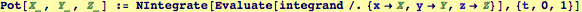We will create contourplots (level sets of constant potential) at as a function of different heights. We check the timing of the computation to compare to method in the last lecture.

In:=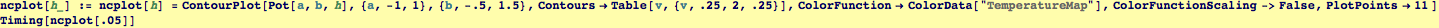Out=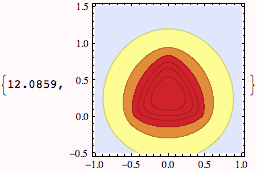In:=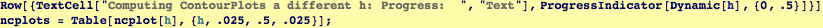Out=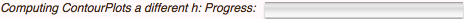In:=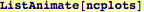Out=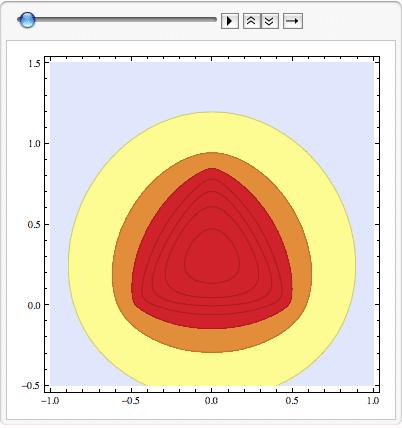Created by Wolfram Mathematica 6.0  (28 September 2007)